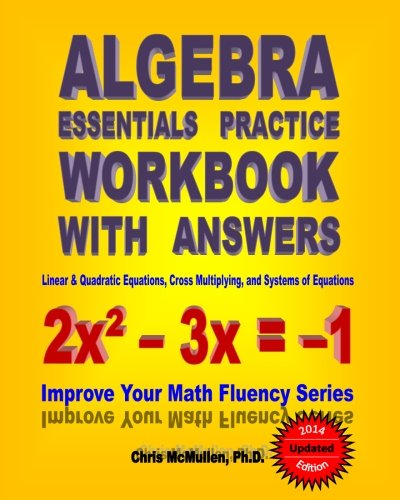Please Enter ISBN, Title or Author’s Nameand more...

# Algebra Essentials Practice Workbook with Answers: Linear & Quadratic Equations, Cross Multiplying, and Systems of Equations: Improve Your Math Fluency SeriesAuthor: Chris McMullen
ISBN:1453661387
ISBN-13: 9781453661383
List Price: \$9.99 (up to 65% savings)
Prices shown are the lowest from
the top textbook retailers.

## View all Prices by Retailer## Details about Algebra Essentials Practice Workbook with Answers: Linear & Quadratic Equations, Cross Multiplying, and Systems of Equations: Improve Your Math Fluency Series:

AUTHOR: Chris McMullen earned his Ph.D. in physics from Oklahoma State University and currently teaches physics at Northwestern State University of Louisiana. He developed the Improve Your Math Fluency series of workbooks to help students become more fluent in basic math skills.

CONTENTS: This Algebra Essentials Practice Workbook with Answers provides ample practice for developing fluency in very fundamental algebra skills – in particular, how to solve standard equations for one or more unknowns. These algebra 1 practice exercises are relevant for students of all levels – from grade 7 thru college algebra. This workbook is conveniently divided up into seven chapters so that students can focus on one algebraic method at a time. Skills include solving linear equations with a single unknown (with a separate chapter dedicated toward fractional coefficients), factoring quadratic equations, using the quadratic formula, cross multiplying, and solving systems of linear equations. Not intended to serve as a comprehensive review of algebra, this workbook is instead geared toward the most essential algebra skills. An introduction describes how parents and teachers can help students make the most of this workbook. Students are encouraged to time and score each page. In this way, they can try to have fun improving on their records, which can help lend them confidence in their math skills.

PRACTICE: With no pictures, this workbook is geared strictly toward learning the material and developing fluency through practice.

EXAMPLES: Each section begins with a few pages of instructions for how to solve the equations followed by a few examples. These examples should serve as a useful guide until students are able to solve the problems independently.

ANSWERS: Answers to exercises are tabulated at the back of the book. This helps students develop confidence and ensures that students practice correct techniques, rather than practice making mistakes.

PHOTOCOPIES: The copyright notice permits parents/teachers who purchase one copy or borrow one copy from a library to make photocopies for their own children/students only. This is very convenient if you have multiple children/students or if a child/student needs additional practice.

Need Elementary tutors? Start your search below:
Need Elementary course notes? Start your search below: